• +91 9971497814
• info@interviewmaterial.com

# RD Chapter 10- Congruent Triangles Ex-10.4 Interview Questions Answers

### Related Subjects

Question 1 : In the figure, AB || CD and ∠1 and ∠2 are in the ratio 3 : 2. Determine all angles from 1 to 8.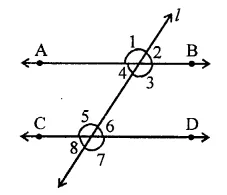AB || CD and l is transversal ∠1 : ∠2 = 3 : 2
Let ∠1 = 3x
Then ∠2 = 2x
But ∠1 + ∠2 = 180° (Linear pair)
∴ 3x + 2x = 180° ⇒ 5x = 180°
⇒ x =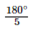= 36°
∴ ∠1 = 3x = 3 x 36° = 108°
∠2 = 2x = 2 x 36° = 72°
Now ∠1 = ∠3 and ∠2 = ∠4 (Vertically opposite angles)
∴ ∠3 = 108° and ∠4 = 72°
∠1 = ∠5 and ∠2 = ∠6 (Corresponding angles)
∴ ∠5 = 108°, ∠6 = 72°
Similarly, ∠4 = ∠8 and
∠3 = ∠7
∴ ∠8 = 72° and ∠7 = 108°
Hence, ∠1 = 108°, ∠2= 72°
∠3 = 108°, ∠4 = 72°
∠5 = 108°, ∠6 = 72°
∠7 = 108°, ∠8 = 12°

Question 2 : In the figure, l, m and n are parallel lines intersected by transversal p at X, Y and Z respectively. Find ∠l, ∠2 and ∠3.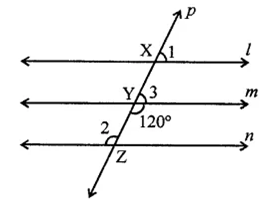l || m || n and p is then transversal which intersects then at X, Y and Z respectively ∠4 = 120°
∠2 = ∠4 (Alternate angles)
∴ ∠2 = 120°
But ∠3 + ∠4 = 180° (Linear pair)
⇒ ∠3 + 120° = 180°
⇒ ∠3 = 180° – 120°
∴ ∠3 = 60°
But ∠l = ∠3 (Corresponding angles)
∴ ∠l = 60°
Hence ∠l = 60°, ∠2 = 120°, ∠3 = 60°

Question 3 : In the figure, if AB || CD and CD || EF, find ∠ACE.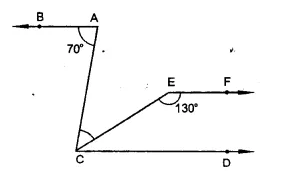Given : In the figure, AB || CD and CD || EF
∠BAC = 70°, ∠CEF = 130°
∵ EF || CD
∴ ∠ECD + ∠CEF = 180° (Co-interior angles)
⇒ ∠ECD + 130° = 180°
∴ ∠ECD = 180° – 130° = 50°
∵ BA || CD
∴ ∠BAC = ∠ACD (Alternate angles)
∴ ∠ACD = 70° (∵ ∠BAC = 70°)
∵ ∠ACE = ∠ACD – ∠ECD = 70° – 50° = 20°

Question 4 : In the figure, state which lines are parallel and why.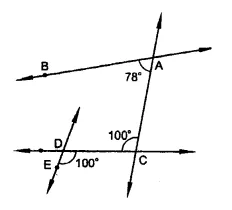In the figure,
∵ ∠ACD = ∠CDE = 100°
But they are alternate angles
∴ AC || DE

Question 5 : In the figure, if l || m,n|| p and ∠1 = 85°, find ∠2.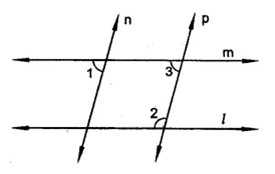In the figure, l || m, n|| p and ∠1 = 85°
∵ n || p
∴ ∠1 = ∠3 (Corresponding anlges)
But ∠1 = 85°
∴ ∠3 = 85°
∵ m || 1
∠3 + ∠2 = 180° (Sum of co-interior angles)
⇒ 85° + ∠2 = 180°
⇒ ∠2 = 180° – 85° = 95°

Question 6 : If two straight lines are perpendicular to the same line, prove that they are parallel to each other.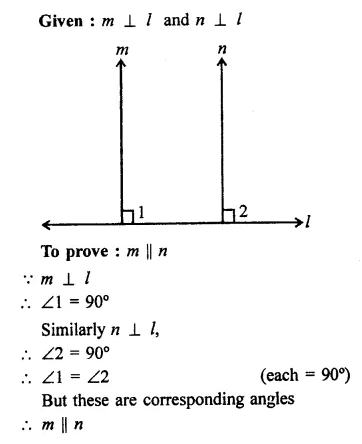Question 7 : Two unequal angles of a parallelogram are in the ratio 2:3. Find all its angles in degrees.

In ||gm ABCD,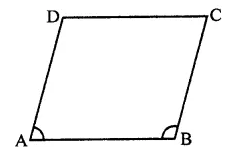∠A and ∠B are unequal
and ∠A : ∠B = 2 : 3
Let ∠A = 2x, then
∠B = 3x
But ∠A + ∠B = 180° (Co-interior angles)
∴ 2x + 3x = 180°
⇒ 5x = 180°
⇒ x == 36°
∴ ∠A = 2x = 2 x 36° = 72°
∠B = 3x = 3 x 36° = 108°
But ∠A = ∠C and ∠B = ∠D (Opposite angles of a ||gm)
∴ ∠C = 72° and ∠D = 108°
Hence ∠A = 72°, ∠B = 108°, ∠C = 72°, ∠D = 108°

Question 8 : In each of the two lines is perpendicular to the same line, what kind of lines are they to each other?

AB ⊥ line l and CD ⊥ line l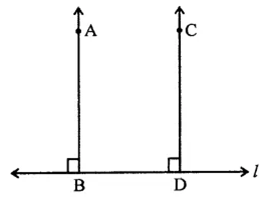∴ ∠B = 90° and ∠D = 90°
∴ ∠B = ∠D
But there are corresponding angles
∴ AB || CD

Question 9 : In the figure, ∠1 = 60° and ∠2 = (23)3 a right angle. Prove that l || m.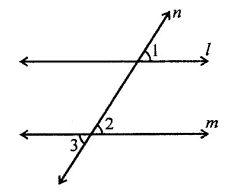In the figure, a transversal n intersects two lines l and m
∠1 = 60° and
∠2 =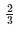rd of a right angle 2
=x 90° = 60°
∴ ∠1 = ∠2
But there are corresponding angles
∴ l || m

Question 10 : In the figure, if l || m || n and ∠1 = 60°, find ∠2.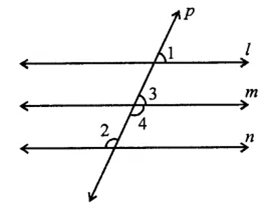In the figure,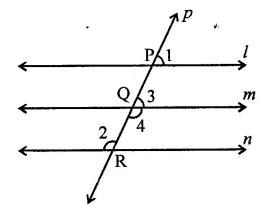l || m || n and a transversal p, intersects them at P, Q and R respectively
∠1 = 60°
∴ ∠1 = ∠3 (Corresponding angles)
∴ ∠3 = 60°
But ∠3 + ∠4 = 180° (Linear pair)
60° + ∠4 = 180° ⇒ ∠4 = 180° – 60°
∴ ∠4 = 120°
But ∠2 = ∠4 (Alternate angles)
∴ ∠2 = 120°

Todays Deals### RD Chapter 10- Congruent Triangles Ex-10.4 Contributorskrishan

Name:
Email:

# Latest News# 9000 interview questions in different categories